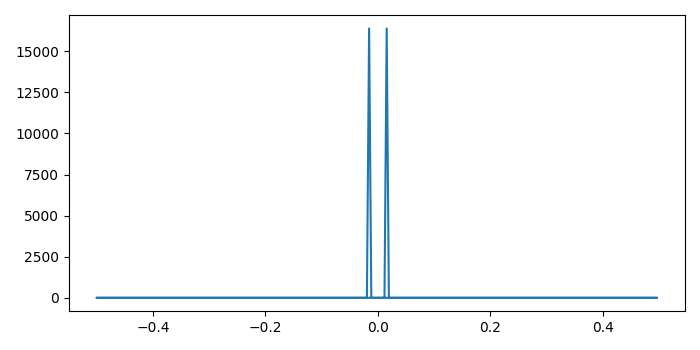# Matplotlib – How to plot the FFT of signal with correct frequencies on the X-axis?

MatplotlibPythonData Visualization

To plot the FFT (Fast Fourier Transform) of a signal with correct frequencies on the X-axis in matplotlib, we can take the following steps −

## Steps

• Set the figure size and adjust the padding between and around the subplots.

• Initialize two variables, N and m, to calculate nu.

• Create the signal (a sine wave) using numpy. Compute the one-dimensional discrete Fourier Transform.

• Return the Discrete Fourier Transform sample frequencies.

• Plot the freq and fourier transform data points.

• To display the figure, use Show() method.

## Example

import numpy as np
import matplotlib.pyplot as plt

plt.rcParams["figure.figsize"] = [7.00, 3.50]
plt.rcParams["figure.autolayout"] = True

N = 256
t = np.arange(N)
m = 4
nu = float(m)/N

signal = np.sin(2*np.pi*nu*t)

ft = np.fft.fft(signal)

freq = np.fft.fftfreq(N)

plt.plot(freq, ft.real**2 + ft.imag**2)

plt.show()


## Output

It will produce the following output −